### Choose language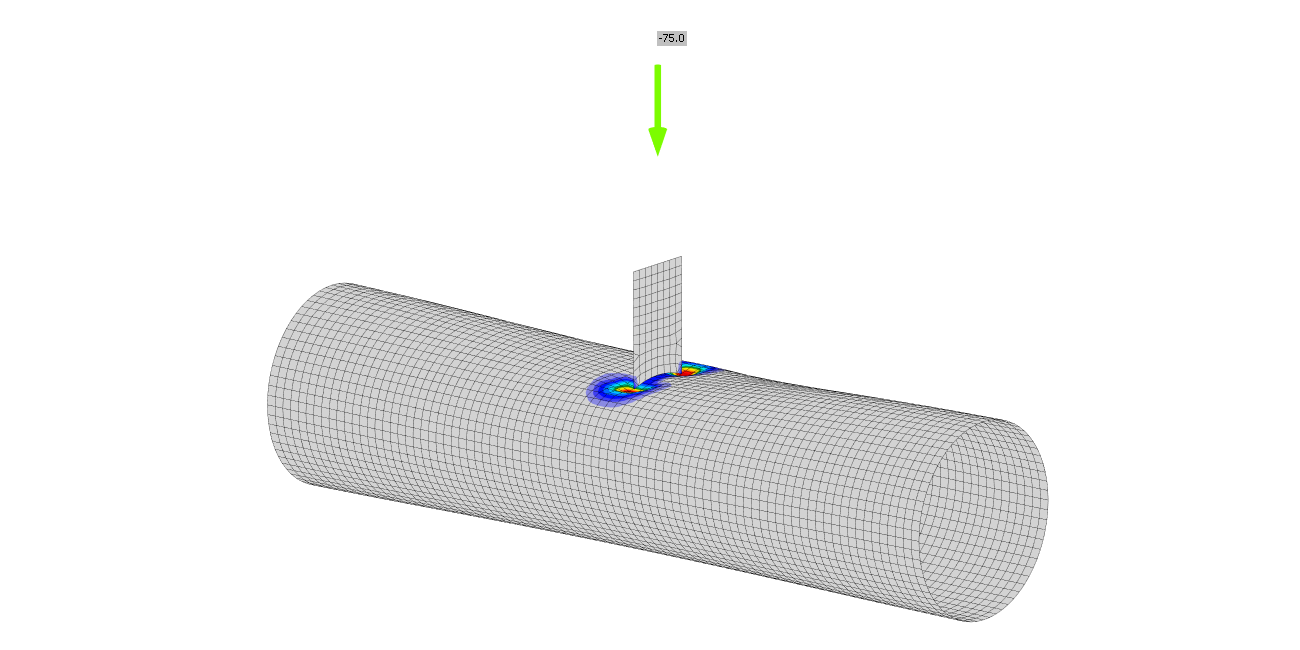# Plate to circular hollow section

This is a selected chapter from book Component-based finite element design of steel connections by prof. Wald et al. The chapter is focused on verification of connection of plate to circular hollow section.
$$Failure mode methodUniplanar welded plate to circular hollow sections T-joints predicted by CBFEM are verified to FMM in this chapter. In CBFEM, the design resistance is limited by reaching 5 % of strain or a force corresponding to 3 % d0 joint deformation, where d0 is chord diameter. The FMM is based on the peak load limit or 3 % d0 deformation limit; see Lu et al. (1994). The welds, designed according to EN 1993‑1‑8:2006, are not the weakest components in the joint.Chord plastificationThe design resistance of a CHS chord face is determined using the method given by FMM model in Ch. 9 of prEN 1993-1-8:2020 and in ISO/FDIS 14346; see Fig. 7.3.1. The design resistance of the axially loaded welded plate to CHS joint is:T jointTransverse$N_{1,Rd} = 2.5 C_f f_{y0} t_0^2 (1+3 \beta^2) \gamma^{0.35} Q_f / \gamma_{M5}$Longitudinal$N_{1,Rd} = 7.4 C_f f_{y0} t_0^2 (1+0.4 \eta) Q_f / \gamma_{M5}$X jointTransverse$N_{1,Rd} = 2.1 C_f f_{y0} t_0^2 (1+3 \beta^2) \gamma^{0.25} Q_f / \gamma_{M5}$Longitudinal$N_{1,Rd} = 3.5 C_f f_{y0} t_0^2 (1+0.4 \eta^2) \gamma^{0.1} Q_f / \gamma_{M5}$where: fy,i – yield strength of member i (i = 0,1,2 or 3) ti – thickness of the wall of CHS member i (i = 0,1,2 or 3) $$\beta$$ – ratio of the mean diameter or width of brace members, to that of the chord $$\eta$$ – ratio of the brace member depth to the chord diameter or width $$\gamma$$ – ratio of a chord width or diameter to twice its wall thickness Qf – chord stress factor Cf – material factor $$\gamma_{M5}$$ – partial factor for resistance of joints in hollow section lattice girders Ni,Rd – design resistance of a joint expressed in terms of the internal axial force in member i (i = 0,1,2 or 3)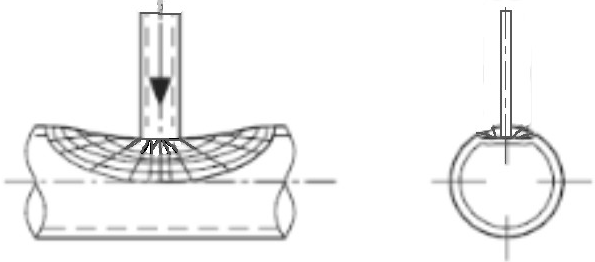Fig. 7.3.1 Examined failure mode - chord plastificationRange of validityCBFEM was verified for typical joints of the welded circular hollow sections. The range of validity for these joints is defined in Table 7.8 of prEN 1993-1-8:2020; see Tab 7.3.1. The same range of validity is applied to CBFEM model. Outside the range of validity of FMM, an experiment should be prepared for validation or verification performed for verification according to a validated research model.Tab. 7.3.1 Range of validity for method of failure modes General$$0.2 \le \frac{d_i}{d_0} \le 1.0$$$$\theta_i \ge 30^{\circ}$$$$-0.55 \le \frac{e}{d_0} \le 0.25$$ $$g \ge t_1+t_2$$$$f_{yi} \le f_{y0}$$$$t_i \le t_0$$ ChordCompressionClass 1 or 2 and $$10 \le d_0 / t_0 \le 50$$ (but for X joints: $$d_0/t_0 \le 40$$) Tension$$10 \le d_0 / t_0 \le 50$$ (but for X joints: $$d_0/t_0 \le 40$$) Transverse plate$$0.25\le\beta=b_1/d_0\le1$$ Longitudinal plate$$0.6\le\eta=h_1/d_0\le4$$ ValidationIn this chapter, the CBFEM is validated to the FMM models of plate to CHS T-joints described in prEN 1993-1-8:2020. The models are compared against the data from mechanical tests in Tabs 7.3.2–7.3.3 with resistance based on deformation limit. Material and geometric properties of numerical tests are described in (Voth A.P. and Packer A.J., 2010). The experiments out of range of validity are marked in tables by star * and in graph indicated to show the quality of the boundary conditions.Tab. 7.3.2 Geometric properties, material properties, and resistances of connections from experiments and FMM models for transverse T-joint IDReferenced0 [mm] t0 [mm] h1 [mm] h1/d0 [-] d0/t0 [-] fy0 [MPa] TPT 1Washio et al. (1970)165,25,2115,60,731,8308,0 TPT 2Washio et al. (1970)165,25,2148,70,931,8308,0 TPT 3Washio et al. (1970)139,83,5125,80,939,9343,0 TPT 4Voth et al. (2012)219,24,5100,30,548,8388,8 IDNu,exp [kN] Branch typeNu,exp/(t02·fy0)N1,prEN/(t02·fy0)Nu,exp/N1,prEN TPT 1169,4Compression20,3416,251,25 TPT 2250,5Compression30,0822,581,33 TPT 3184,8Compression43,9824,451,80 TPT 4282,5Tension36,0412,452,89 Tab. 7.3.3 Geometric properties, material properties, and resistances of connections from experiments and FMM models for longitudinal T-joint IDReferenced0 [mm] t0 [mm] h1 [mm] h1/d0 [-] d0/t0 [-] fy0 [MPa] TPL 1Washio et al. (1970)165,25,2165,21,031,8308,0 TPL 2Washio et al. (1970)165,25,2330,42,031,8308,0 *TPL 3Voth et al. (2012)219,24,599,90,548,8388,8 IDNu,exp [kN] Branch typeNu,exp/(t02·fy0)N1,prEN/(t02·fy0)Nu,exp/N1,prEN TPL 1107,6Compression12,9210,361,25 TPL 2127,4Compression15,3013,321,15 *TPL 3160,6Tension20,498,752,34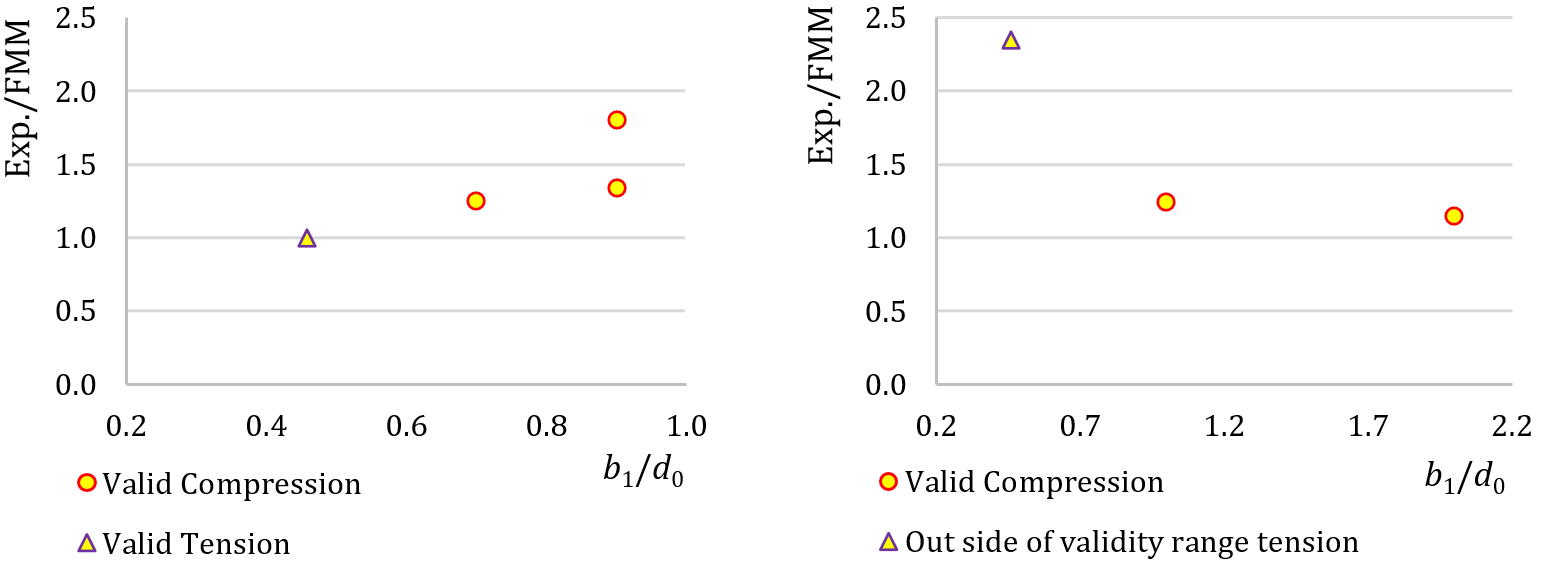Fig. 7.3.2 Validation of FMM to mechanical experiments for transverse T-type plate-to-CHS connections (left) and to longitudinal T-type plate-to-CHS connections (right)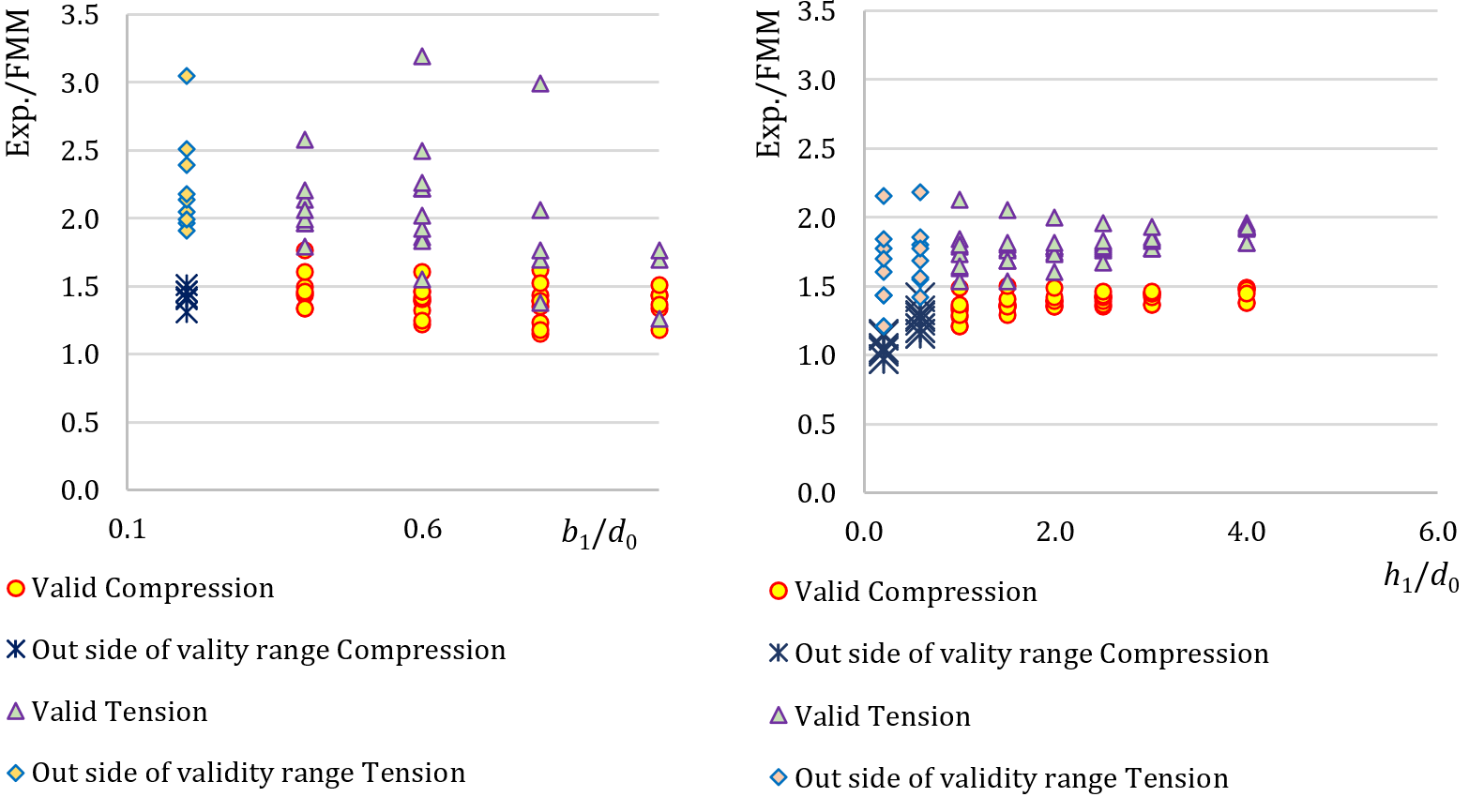Fig. 7.3.3 Validation of FMM to mechanical experiments for transverse T-type plate-to-CHS connections (left) and longitudinal T-type plate-to-CHS connections (right)The validation shown in Figs 7.3.2 and 7.3.3 demonstrates that differences to experiments are at least 15 % generally to the safe side. The experiments out of the range of validity are included and marked. The results indicate the good quality of the chosen boundary conditions.Uniplanar plate T-jointAn overview of the considered examples in the study is given in the Tab. 7.3.4. Selected cases cover a wide range of joint geometric ratios. Geometry of the joints with dimensions is shown in Fig. 7.3.4. Plate thickness is 15 mm in all cases covered in this study.Tab. 7.3.4 Examples overview ExampleChordPlate orientationPlate widthMaterial Section-b1/h1fyfuE [mm][MPa][MPa][GPa] 1CHS219,1/4,5Transverse80355490210 2CHS219,1/5,0Transverse80355490210 3CHS219,1/4,5Longitudinal180355490210 4CHS219,1/4,5Longitudinal250355490210 5CHS219,1/4,5Longitudinal380355490210 6CHS219,1/4,5Longitudinal500355490210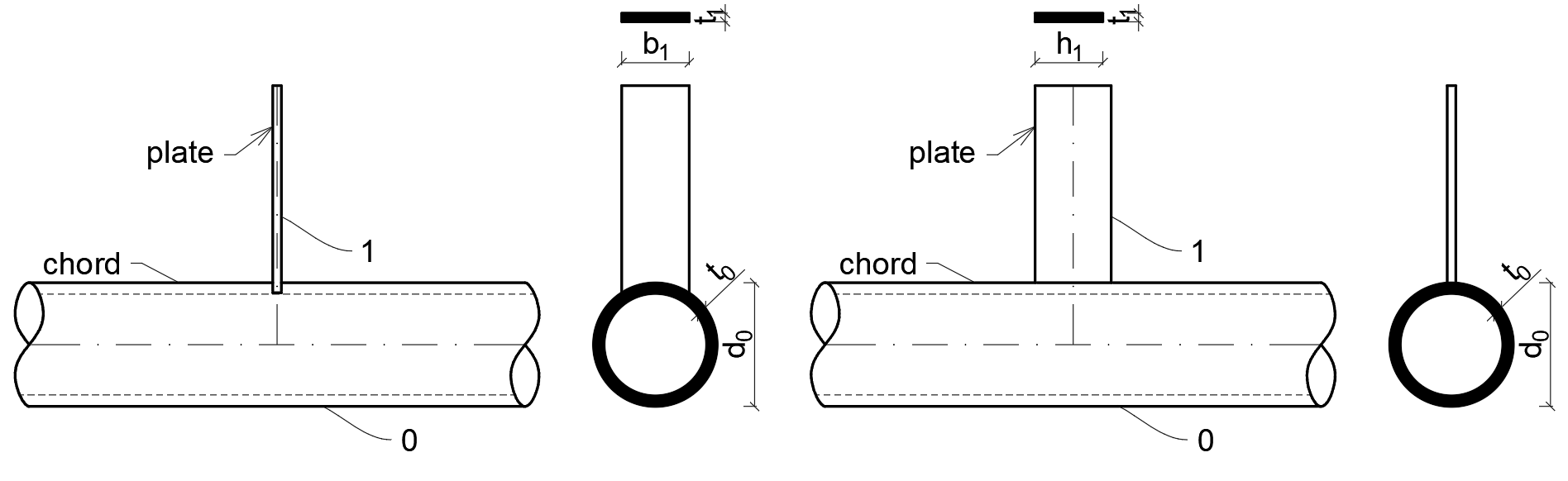Fig. 7.3.4 Dimensions of plate to CHS T joint, transverse (left) and longitudinal (right)VerificationThe results of resistance and design failure mode of the FMM are compared with the results of CBFEM in Tab. 7.3.5 and in Fig. 7.3.5.Tab. 7.3.5 Verification of prediction of resistances by CBFEM on FMM ExampleFMM Mode of failureCBFEMMode of failurediff. [kN] [kN] [%] 176,9Chord plastification77,3Chord plastification0,5 291,5Chord plastification90,6Chord plastification1,0 370,7Chord plastification65,2Chord plastification8,4 477,5Chord plastification71,9Chord plastification7,8 590,1Chord plastification82,8Chord plastification8,8 6101,8Chord plastification93,0Chord plastification9,4 The study shows good agreement for the applied load cases. The results are summarized in a diagram comparing CBFEM’s and FMM’s design resistances; see Fig. 7.3.5. The results show that the difference between the two calculation methods is in all cases less than 7 %.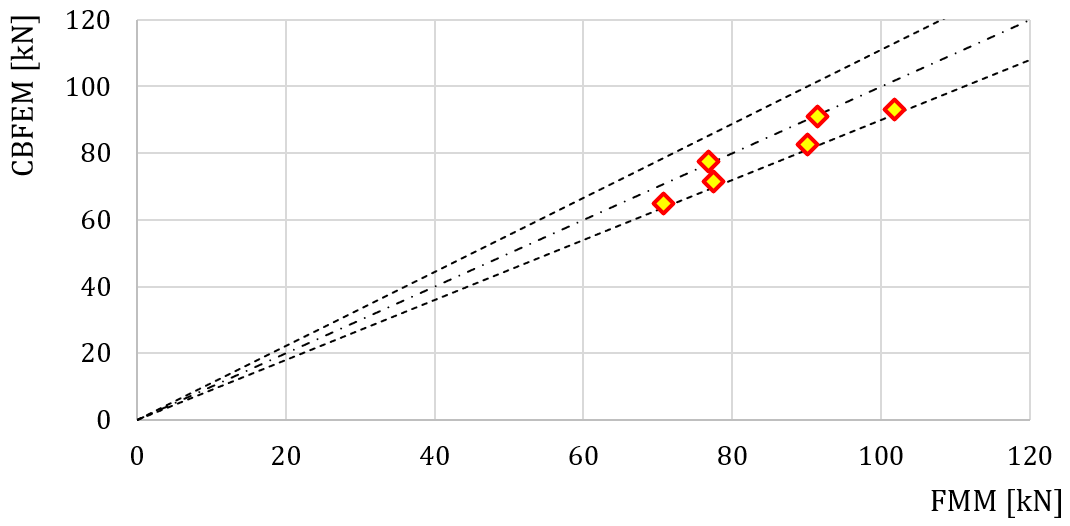Fig. 7.3.5 Verification of CBFEM to FMM for the uniplanar Plate to CHS T-jointBenchmark exampleInputsChord Steel S355 Section CHS219.1/4,5 Brace Steel S355 Plate 80/15 mm Angle between the brace member and the chord 90° (transversal) Weld Butt weld around the brace Loaded By force to brace in compression Mesh size 64 elements along surface of the circular hollow member Outputs The design resistance in compression is NRd = 77,3 kN The design failure mode is chord plastification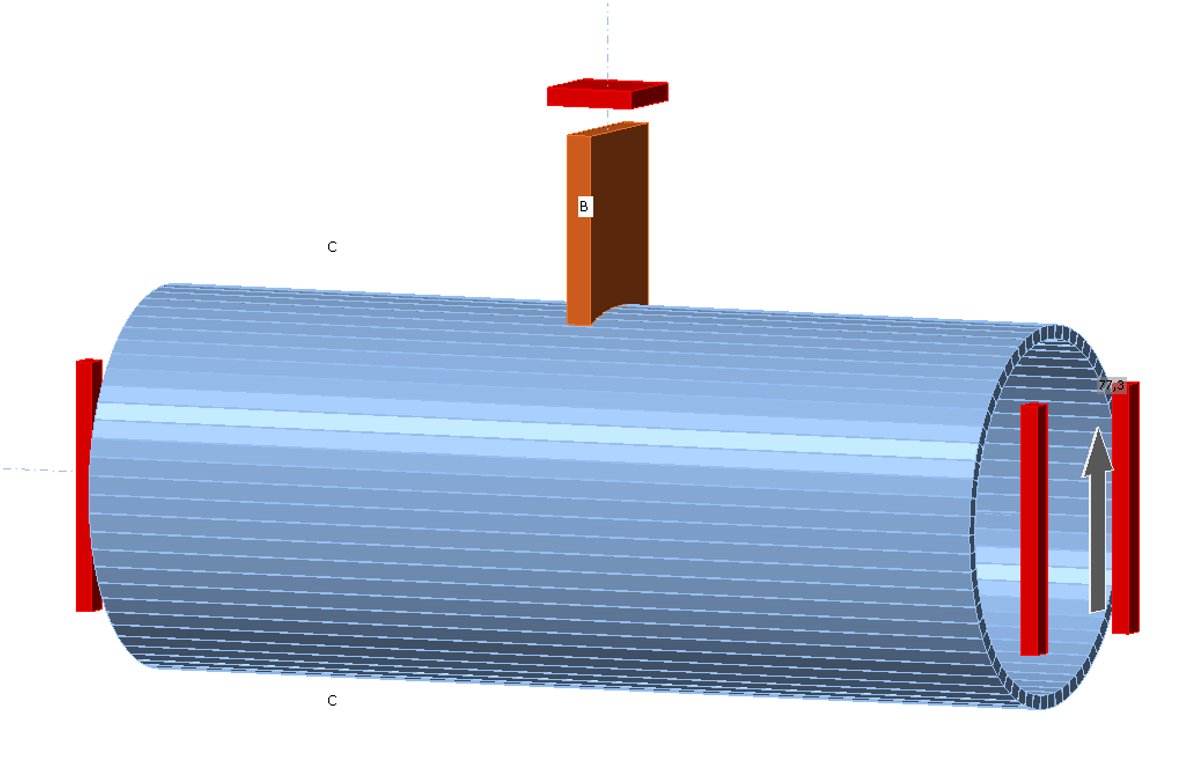Fig. 7.3.6 Boundary conditions for the uniplanar Plate to CHS T-joint Sample files Open in Viewer Download$$